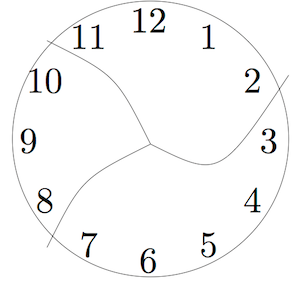$$\newcommand{\id}{\mathrm{id}}$$ $$\newcommand{\Span}{\mathrm{span}}$$ $$\newcommand{\kernel}{\mathrm{null}\,}$$ $$\newcommand{\range}{\mathrm{range}\,}$$ $$\newcommand{\RealPart}{\mathrm{Re}}$$ $$\newcommand{\ImaginaryPart}{\mathrm{Im}}$$ $$\newcommand{\Argument}{\mathrm{Arg}}$$ $$\newcommand{\norm}{\| #1 \|}$$ $$\newcommand{\inner}{\langle #1, #2 \rangle}$$ $$\newcommand{\Span}{\mathrm{span}}$$

# 1.8: The Last Step

•• Contributed by Michelle Manes
• Professor (Mathematics) at University of Hawaii
$$\newcommand{\vecs}{\overset { \rightharpoonup} {\mathbf{#1}} }$$ $$\newcommand{\vecd}{\overset{-\!-\!\rightharpoonup}{\vphantom{a}\smash {#1}}}$$$$\newcommand{\id}{\mathrm{id}}$$ $$\newcommand{\Span}{\mathrm{span}}$$ $$\newcommand{\kernel}{\mathrm{null}\,}$$ $$\newcommand{\range}{\mathrm{range}\,}$$ $$\newcommand{\RealPart}{\mathrm{Re}}$$ $$\newcommand{\ImaginaryPart}{\mathrm{Im}}$$ $$\newcommand{\Argument}{\mathrm{Arg}}$$ $$\newcommand{\norm}{\| #1 \|}$$ $$\newcommand{\inner}{\langle #1, #2 \rangle}$$ $$\newcommand{\Span}{\mathrm{span}}$$ $$\newcommand{\id}{\mathrm{id}}$$ $$\newcommand{\Span}{\mathrm{span}}$$ $$\newcommand{\kernel}{\mathrm{null}\,}$$ $$\newcommand{\range}{\mathrm{range}\,}$$ $$\newcommand{\RealPart}{\mathrm{Re}}$$ $$\newcommand{\ImaginaryPart}{\mathrm{Im}}$$ $$\newcommand{\Argument}{\mathrm{Arg}}$$ $$\newcommand{\norm}{\| #1 \|}$$ $$\newcommand{\inner}{\langle #1, #2 \rangle}$$ $$\newcommand{\Span}{\mathrm{span}}$$

A lot of people — from Polya to the writers of the Common Core State Standards and a lot of people in between — talk about problem solving in mathematics. One fact is rarely acknowledged, except by many professional mathematicians: Asking good questions is as valuable (and as difficult) as solving mathematical problems.

After solving a mathematical problem and explaining your solution to someone else, it is a very good mathematical habit to ask yourself: What other questions can I ask?

Example $$\PageIndex{1}$$: Squares on a Chess Board

Recall Problem 3, “Squares on a Chess Board”:

How many squares of any possible size are on a standard 8 × 8 chess board? (The answer is not 64! It’s a lot bigger!)

We have already talked about some obvious follow-up questions like “What about a 10 × 10 chess board? Or 100 × 100? Or $$n \times n$$?”

But there are a lot of interesting (and less obvious . . . and harder) questions you might ask:

• How many rectangles of any size and shape can you find on a standard 8 × 8 chess board? (This is a lot harder, because the rectangles come in all different sizes, like 1 × 2 and 5 × 3. How could you possibly count them all?)
• How many triangles of any size and shape can you find in this picture?Example $$\PageIndex{2}$$: Broken Clock

Recall Problem 4, “Broken Clock”:

This clock has been broken into three pieces. If you add the numbers in each piece, the sums are consecutive numbers. Can you break another clock into a different number of pieces so that the sums are consecutive numbers?The original problem only asks if you can find one other way. The obvious follow-up question: “Find every possibly way to break the clock into some number of pieces so that the sums of the numbers on each piece are consecutive numbers. Justify that you have found every possibility.”

Think / Pair / Share

Choose a problem from the Problem Bank (preferably a problem you have worked on, but that is not strictly necessary). What follow-up or similar questions could you ask?